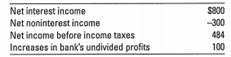### Create an Account

Home / Questions / You were informed the banks latest income and expense statement contained the following fi...

# You were informed the banks latest income and expense statement contained the following figures in \$ millions Suppose you also were told that the bank& 39 s total interest income is twice as

You were informed the bank’s latest income and expense statement contained the following figures (in \$ millions ) ?Suppose you also were told that the bank&#39;s total interest income is twice as large as its total interest expense and its noninterest income is three-fourths of its noninterest expense. Imagine that its provision for loan losses equals 1 percent of its total interest income, while its taxes generally amount to 30 percent of its net income before income taxes. Calculate the following items for this bank&#39;s income and expense statement:

Apr 24 2020 View more View LessSubscribe To Get Solution